### Symmetry - Worksheets

CBSE Worksheet 01

Ch-13 Symmetry
1. Find the number of lines of symmetry in the given below:1. None of these
2. 4
3. 2
4. 3
2. If a figure has two or more lines of symmetry, should it have rotational symmetry of order more than 1?
1. Yes
2. May be
3. None of these
4. No
3. Which of the followings has no line of symmetry?
1. U
2. H
3. S
4. A
4. The order of the rotational symmetry of the given figure below about the point marked ‘x’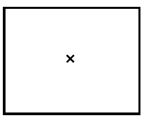1. 1
2. 4
3. 3
4. 0
5. Which of the following figures does not have line of symmetry always?
1. A triangle
2. An angle
3. A square
4. A line
6. Match the following-

Column AColumn B
a. Taj Mahalp. Horizontal & Vertical
b. Scissorq. Symmetric Monument
c. Letter ‘I’r. No line of symmetry
d. Scalene triangles. One line of symmetry
7. Fill in the blanks-

1. The geometrical figure _______ has 2 lines of symmetry.
2. The alphabet ‘A’ has ______ line of symmetry.
3. The circle has _____ line of symmetry.
4. The flower which has lines of symmetry is ______.
8. State whether the following statements are true or false

1. Number ‘zero’ has no line of symmetry.
2. Alphabet ‘Z’ has horizontal line of symmetry.
3. An image in the mirror is as far behind the mirror as the object in front of mirror.
4. Symmetrical objects are identical to each other.
9. Name 2 symmetrical pulses

10. What is the another name of line of symmetry?

11. In each figure alongside, a letter of the alphabet is shown along with a vertical line. Take the mirror image of the letter in the given line. Find which letters look the
same after reflection (i.e., which letters look the same in the image), and which do not. Can you guess why?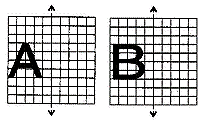Try for O E M N P H L T S V X

12. In the figure, l is the line of symmetry. Draw the image of the triangle and complete the diagram so that it becomes symmetric.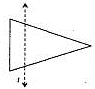13. Draw 3-shape which is not polygon. Draw line of symmetry in it.

14. In environment butterfly are found colourful butterfly are the gift of nature. Does all butterfly have the line of symmetry?

15. What is symmetry? Name all type of symmetry.

CBSE Worksheet 01
Ch-13 Symmetry

1. None of these
Explanation: Zero lines of symmetry exists for this figure.
1. Yes
Explanation: If a figure has two or more lines of symmetry, then it will definitely have its rotational symmetry of order more than 1.
1. S
Explanation: No vertical, horizontal or slanting line on this figure will create parts that are mirror images of each other.
1. 4
Explanation: When rotated 360o, same image of square appears four times.
1. A triangle
Explanation: The scalene triangle has no lines of symmetry.
1. - q
2. - s
3. - p
4. - r
1. Rectangle or Rhombus
2. Vertical-One
3. Infinite
4. Sunflower
1. False
2. False
3. True
4. True
1. Gram and moong

2. Another name of line of symmetry is axis of symmetry.

3. The letters O, M, H, T, V and X look the same after reflections because these letters have 1 vertical line of symmetry.

4.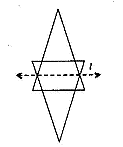1.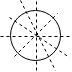2.3.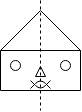5. Yes, all the butterfly have the line of symmetry.
Rough figure and axis of symmetry is as follows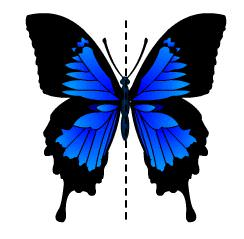6. Symmetry refers to the exact match in shape and size between two halves of an object. It shows the proportional. There are three types of symmetry-

1. Line of symmetry or axis of symmetry

2. Mirror symmetry

3. Rotational symmetry

Line of symmetry or axis of symmetry: A line of symmetry divides a figure in half. Both sides of the figure must be exactly the same.

Mirror symmetry- when you put a mirror along a line of symmetry, the shape formed looks like the original. This type of symmetry is known as reflectional symmetry, eg. cockroach.

Rotational symmetry - When the object is rotated by certain angle, it looks the same and it is termed as rotational symmetry. E.g. fan.